Thursday 04th June 2020
 CBSE Guess > Papers > Question Papers > Class XII > 2010 > Mathematics Mathematics 2010 Question Paper For CBSE Class XII Exams Section A If f: R ?R defined by f(x) = (3 - x3)1/3, then find fof(x). Write the principal value of sec-1(-2). What positive value of X makes the following pair of determinants equal?Evaluate :Write the adjoin of the following matrix :write the value of the following integral.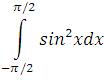A is a square matrix of order 3 and | A| =7. Write the value of |adj . A |. Write the distance of the following plane from the origin : 2 x - y + 2z+1=0 Write a vector of magnitude 9 unites in the direction of vector .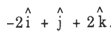Section B A family has 2 children. Find the probability that both are boys, if it is known that (i) At least one of the children is a boy (ii) The elder child is a boy.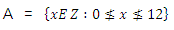Show that the relation S in the set A given by S =is divisible by 4} is an equivalence relation. Find the set of all elements related to 1. Prove the following :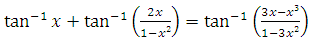Prove the following: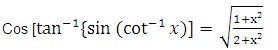Express the following matrix as the sum of a symmetric and skew symmetric matrix, and verify your result :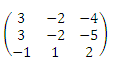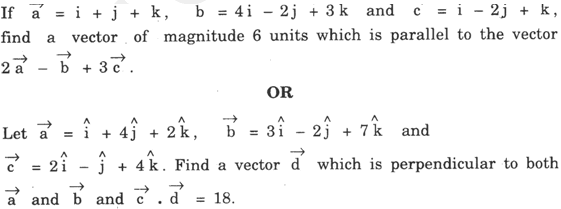Find the points on the line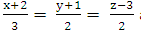at a distance of 5 units from the point P (1, 3, 3).ORFind the distance of the point p(6,5,9) From the plane determined by the points A(3,-1,2 ), B(5,2,4) and C (-1,-1,6). Solve the following differential equation :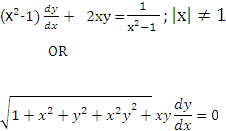Show that the differential equation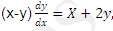is homogeneous and solve it. Evaluate the following ;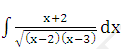Evaluate the following : If y = ea sin-1x , -1 =x =1, then show that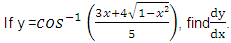Section C Using properties of determinants, prove the following :ORFind the inverse of the following matrix using elementary operations: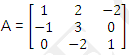A bag contains 4 balls two balls are drawn at random, and are found to be white. What is the probability that all balls are white? One kind of cake requires 300 g of flour and 15 g of fat, another kind of cake requires 150 g of flour and 30 g of fat. Find the maximum number of cakes which can be made from 7.5 kg of flour and 600g of fat, assuming that there is no shortage of the other ingredients' used in making the cakes. Make it as an L.P.P and solve it graphically. Find the coordinates of the foot of the perpendicular and the perpendicular distance of the point p (3, 2, 1) from the plane 2x-y+z+1=0. Find also, the image of the point in the plane. Find the area of the circle 4x² + 4y² =9 which is interior to the parabola x² = 4y.OR Using integration, find the area of the triangle ABC, coordinates of whose vertices are A (4, 1), B (6, 6) and C (8, 4). If the length of three sides of a trapezium other than the base is 10 cm each , find the area of the trapezium, when it is maximum. Find the intervals in which the following function is (a) Strictly increasing (b) Strictly decreasing. Mathematics 2010 Question Papers Class XII Delhi Foreign HTML Format N/A PDF N/A CBSE 2010 Question Papers Class XII